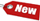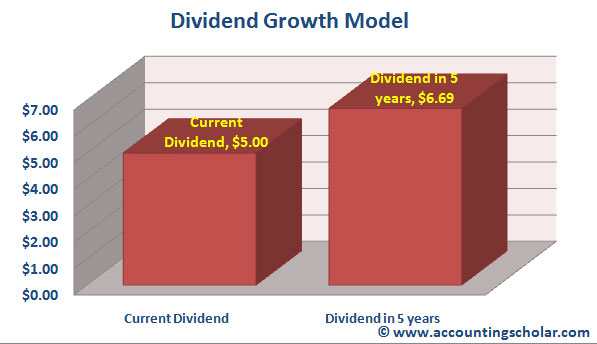Browse Accounting Lessons HereAccounting Terms & DefinitionsAccounting for Merchandising ActivitiesDebits and Credits (Double Entry Accounting)Business Valuation FormulasTime Value of Money & Present/Future ValuesComplex Debt & Equity InstrumentsCommon Stock & Shareholder's EquityAccounting & Finance RatiosValuing Common StockCorporate Income TaxesLower of Cost or Market (LCM) & Inventory ValuationChart of Accounts & BookkeepingBonds Payable & Long Term LiabilitiesCapital AssetsGAAP, Accrual & Cash Accounting, Information Commodity, Internal Controls & MaterialityWhat category of browser are you on this website? Accounting student (homework help) Finance professor (university research) Accounting manager (at work) Other Explore Careers in Accounting and FinanceVisit our section on Careers in Accounting & Finance to explore vast opportunities in this industry.

Dividend Growth Model - How to Value Common Stock with a Constant Dividend and "No Growth"How do we value common stocks for which we know the future prices 2 to more years or periods down the line? For instance, we may be able to estimate what a stock will be worth 2 years from now, and this does not fit our current formula where P1 equals the price of the stock in one year or period. Here’s how to solve this problem. A common stock in a company with a constant dividend is much like a share of preferred stock because the dividend payout does not change. Financial managers also know that the rate of growth on a fixed-rate preferred stock is zero, and thus is constant through time. For a zero growth rate on common stock, thus D1 will be:

 D1 = D2 = D3 = D = Constant

This implies that the dividend payout in Year 2 will be the same as the dividend payout in Year 1, and likewise the dividend payout in Year 3 will be the same as in Year 4, thus D remains constant. Therefore, we can tweak this formula to come up with a new common stock valuation formula:

 P0 = [D / (1 + r)1] + [D / (1 + r)2] + [D / (1 + r)3] + [D / (1 + r)4] + [D / (1 + r)5]

Since the dividend is always the same, the stock can be viewed as an ordinary perpetuity with a cash flow equal to D every period, thus the per-share valuation of the common stock is given by this formula:

 P0 = D / r

Where:

 P0 = Current price of the stock D = Dividend payout at the end of this period r = required rate of return

As an example, consider Cofta Corp. has a policy of paying \$20 per share dividend every year, and the company expects to continue paying out this dividend indefinitely. What will be the value of a common share of stock if the required rate of return is 15%?

 P0 = D / r P0 = \$20 / 0.15 P0 = \$133.33 / share

What if we knew the dividend for this company always grows at a steady rate every year. We will call this growth rate g. If we let D0 be the dividend just paid, then the next dividend D1 will be:

 D1 = D0 x (1 + g)

Where:

 D1 = Value of dividend to be paid next year D0 = Value of dividend paid this year g = Growth rate of dividend

Having said this, what is the formula for dividend payout in 2 periods?

 D2 = D1 x (1 + g)D2 = [D0 x (1 + g) x (1 + g) D2 = D0 x (1 + g)2

We can repeat this process to come up with the dividend at any point in the future. Thus, the dividend Dt in t periods in the future is given by:

 Dt = D0 x (1 + g)t

Example of Dividend Growth

TD Dominion bank has just paid a dividend of \$5 per share and the dividend grows at a steady rate of 6% per year. Based on this information, what will the dividend be in 5 years?

 Dt = D0 x (1 + g)t Dt = \$5 x (1 + 0.06)5 Dt = \$5 x (1 .06)5 Dt = \$5 x 1.3382 Dt = \$6.691

Thus in 5 years, the dividend will grow from \$5 per share to \$6.691 thus growing a total of (\$6.691 - \$5) = \$1.691